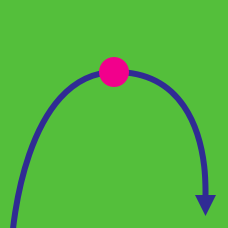Calculus

# Turning Points

Given the function $f(x) = \frac{ x^2-8x + 12}{x^2+6x - 16}$, what is $\displaystyle{\lim_{ x \rightarrow 2 } f(x)}$?

How many integers $k$ are there such that the function $f(x)=x^3+kx^2+3x+2$ has no turning points?

Let $f(x)=x^3-6x^2+14x+9.$ What is the sum of the $x$-coordinates of turning points such that $f(x)$ switches from a decreasing function to an increasing function?

A polynomial of degree $25$ has $m$ real roots and $n$ turning points. What is the maximum value of $m+n$?

What is the sum of all the $x$-coordinates of the turning points in the graph of $f(x)=-2x^3+18x^2-30x+9?$

×